# 30°C to F How to Convert Celsius to Fahrenheit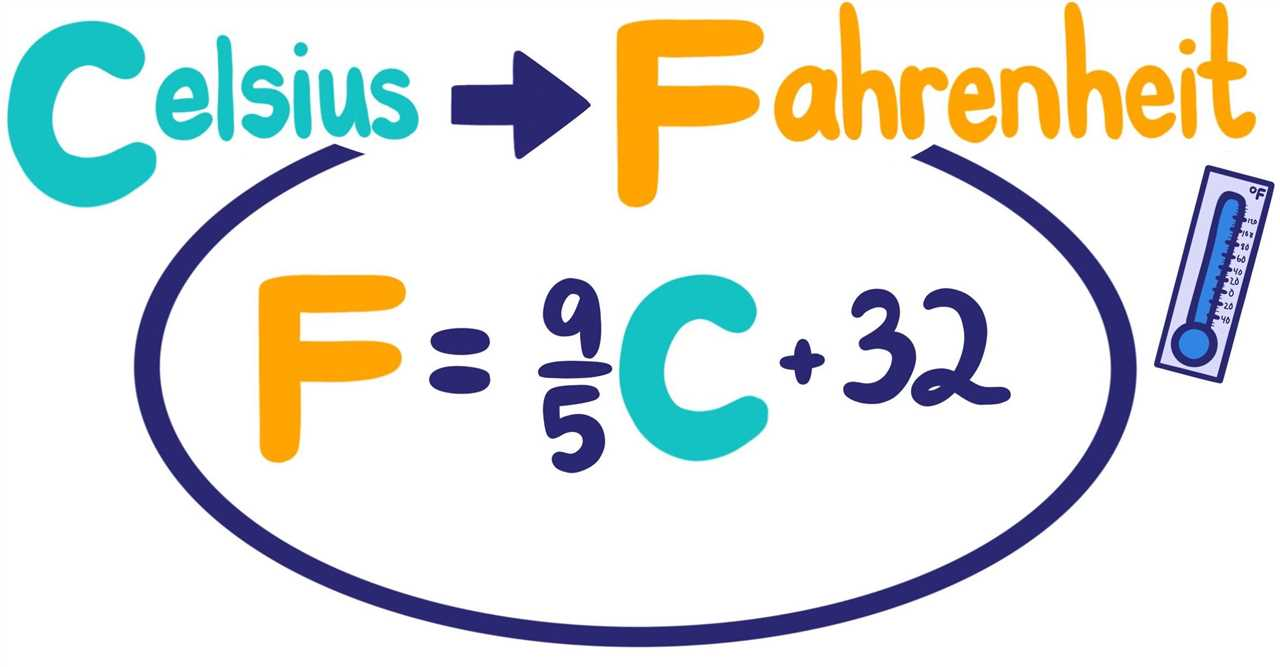Weather forecasts often provide temperatures in Celsius, but if you’re more familiar with Fahrenheit, you may find yourself needing to convert between the two. Fortunately, the conversion from Celsius to Fahrenheit is quite simple and can be done using a simple formula.

The formula to convert Celsius to Fahrenheit is: F = (C * 9/5) + 32. In this formula, F represents the temperature in Fahrenheit, and C represents the temperature in Celsius. By plugging in the Celsius temperature into this formula, you can quickly convert it to Fahrenheit.

For example, if you have a temperature of 30°C and want to convert it to Fahrenheit, you would use the formula: F = (30 * 9/5) + 32. Simplifying this equation gives you F = 86. So, 30°C is equal to 86°F.

If you don’t want to do the math yourself, you can also use an online Celsius to Fahrenheit converter or a calculator. These tools will do the conversion for you and provide you with the equivalent temperature in Fahrenheit.

Converting Celsius to Fahrenheit is a simple process that can be done using a formula or with the help of online tools. Whether you’re trying to figure out the weather for your next vacation or just want to understand temperatures in a different unit, knowing how to convert Celsius to Fahrenheit can be useful in various situations.

## What is Celsius and Fahrenheit?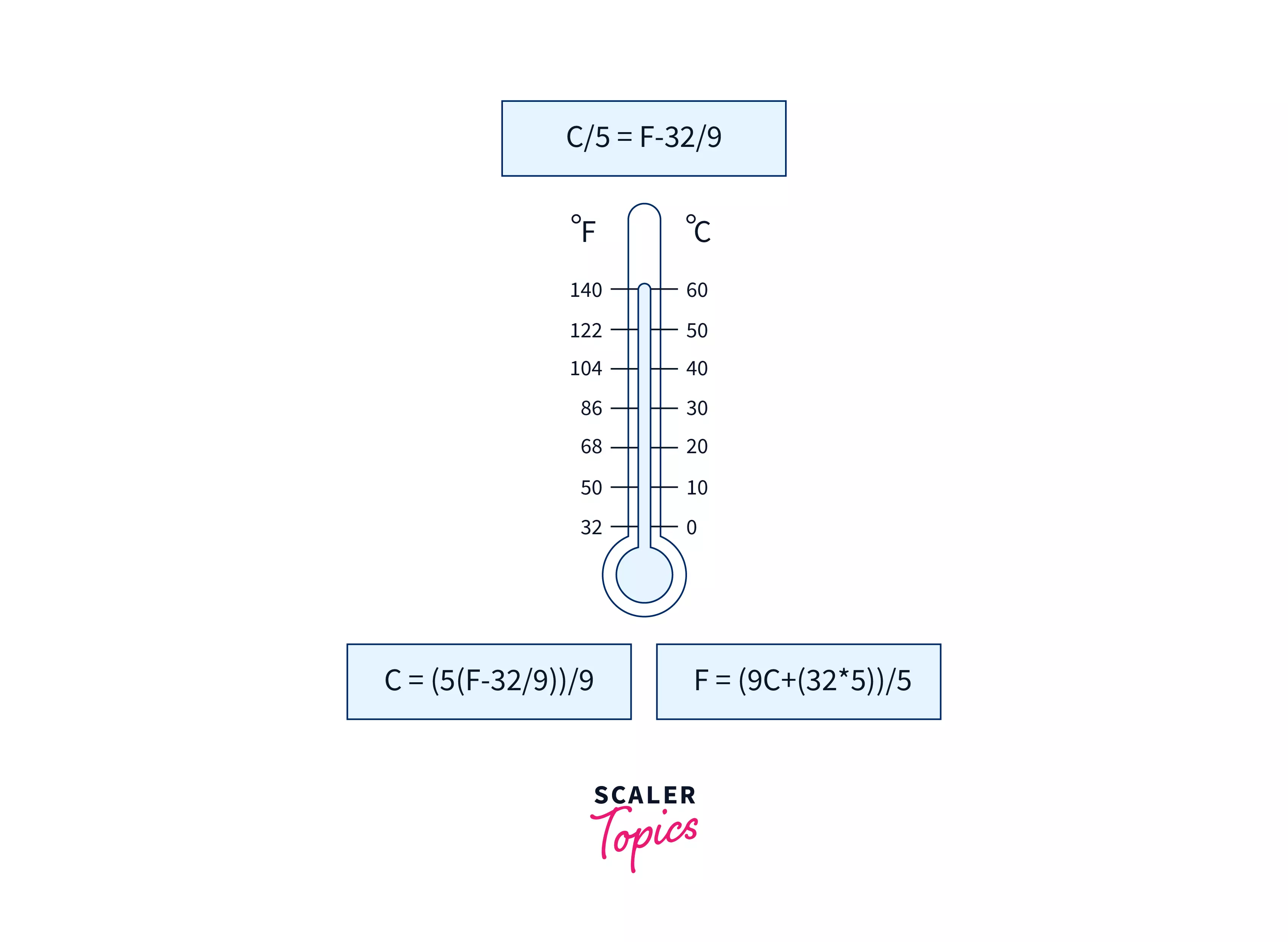Celsius and Fahrenheit are two different temperature scales used to measure temperature. Temperature is a measure of how hot or cold something is.

The Celsius scale is commonly used in most countries around the world, while the Fahrenheit scale is primarily used in the United States and a few other countries. The Celsius scale is based on the freezing and boiling points of water, with 0 degrees Celsius (°C) being the freezing point and 100 degrees Celsius being the boiling point at sea level.

The Fahrenheit scale, on the other hand, is based on a different set of reference points. It was developed by Daniel Gabriel Fahrenheit in the early 18th century. In this scale, the freezing point of water is 32 degrees Fahrenheit (°F) and the boiling point is 212 degrees Fahrenheit at sea level.

To convert temperatures from Celsius to Fahrenheit, you can use the following formula:

Fahrenheit = (Celsius × 9/5) + 32

Similarly, to convert temperatures from Fahrenheit to Celsius, you can use the following formula:

Celsius = (Fahrenheit – 32) × 5/9

Understanding the conversion between Celsius and Fahrenheit is important, especially when dealing with weather forecasts or when traveling to countries that use a different temperature scale. Being able to convert between the two scales allows you to better understand and compare temperatures in different units.

Celsius Fahrenheit
0°C 32°F
10°C 50°F
20°C 68°F
30°C 86°F
40°C 104°F

Remember, when converting temperatures, it’s important to use the correct formula to ensure accurate results. Whether you’re trying to convert Celsius to Fahrenheit or vice versa, knowing the conversion formula will help you determine the equivalent temperature on the other scale.

READ MORE  Expert Tips on How to Clean and Prevent Dust Buildup in Stair Corners

### Understanding Temperature Scales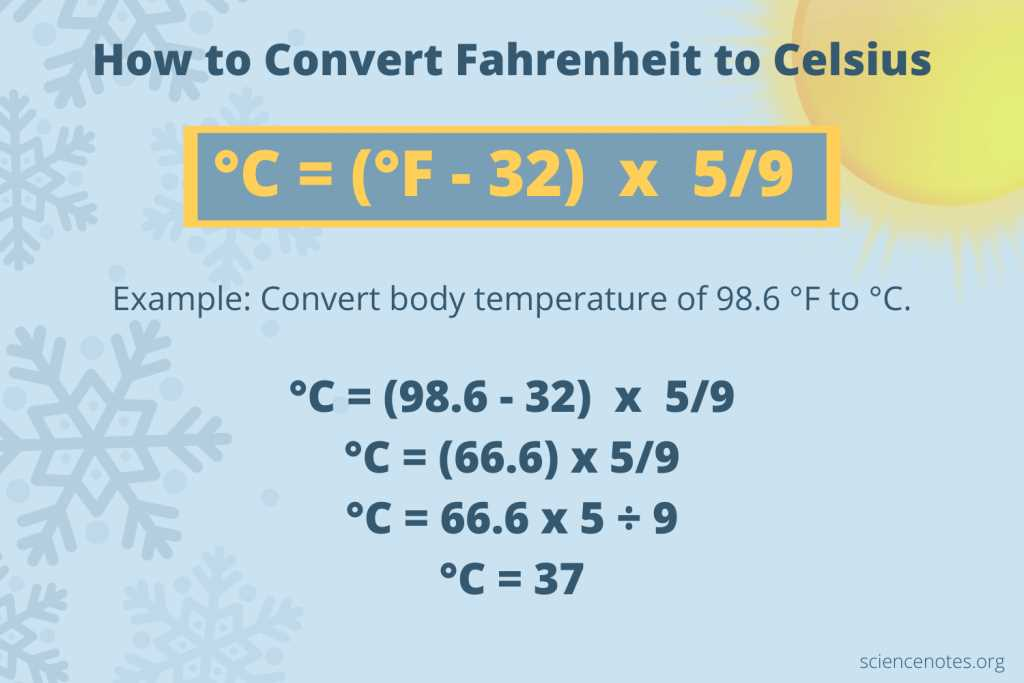Temperature is a measure of the hotness or coldness of an object or environment. It helps us understand the thermal state of things and plays a crucial role in various aspects of our lives, such as weather forecasting, cooking, and scientific research.

There are two commonly used temperature scales: Celsius (°C) and Fahrenheit (°F). While the Celsius scale is widely used worldwide, the Fahrenheit scale is primarily used in the United States and a few other countries.

The Celsius scale is based on the freezing and boiling points of water. On this scale, the freezing point of water is defined as 0 degrees Celsius, while the boiling point of water is defined as 100 degrees Celsius. The Celsius scale is often used in scientific and metric systems.

The Fahrenheit scale, on the other hand, is based on a different set of reference points. The freezing point of water is defined as 32 degrees Fahrenheit, while the boiling point of water is defined as 212 degrees Fahrenheit. The Fahrenheit scale is commonly used in weather forecasts and everyday temperature measurements in the United States.

Converting temperatures from Celsius to Fahrenheit (or vice versa) is a simple process. There is a specific formula to convert a temperature in Celsius to Fahrenheit:

Fahrenheit = (Celsius × 9/5) + 32

For example, to convert 30 degrees Celsius to Fahrenheit, you would use the formula:

Fahrenheit = (30 × 9/5) + 32 = 86 degrees Fahrenheit

Understanding temperature scales is essential for interpreting weather forecasts, comparing temperatures between different regions, and ensuring accurate measurements in various fields. Whether you need to convert Celsius to Fahrenheit or simply want to understand the difference between the two scales, having a grasp of temperature scales is crucial in our daily lives.

### Key Differences Between Celsius and Fahrenheit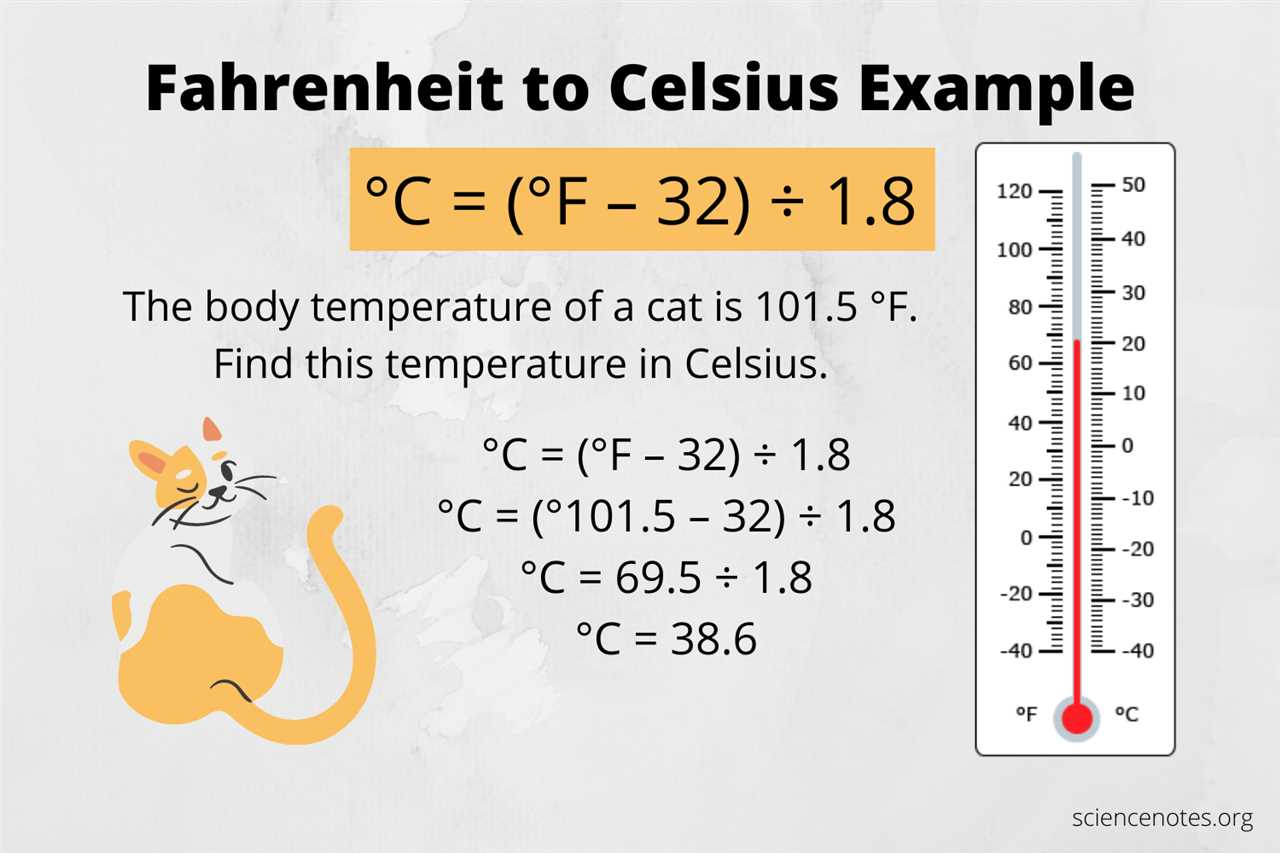The Celsius and Fahrenheit scales are two commonly used temperature scales for measuring temperature. While both scales measure temperature, they have some key differences:

• Celsius: Celsius is the most widely used temperature scale around the world. It is based on the freezing and boiling points of water. On the Celsius scale, the freezing point of water is 0 degrees Celsius, and the boiling point is 100 degrees Celsius.
• Fahrenheit: Fahrenheit is mainly used in the United States and a few other countries. It is based on the freezing and boiling points of water as well, but the scale is different. On the Fahrenheit scale, the freezing point of water is 32 degrees Fahrenheit, and the boiling point is 212 degrees Fahrenheit.
• Conversion: Celsius and Fahrenheit can be converted to each other using specific formulas. To convert Celsius to Fahrenheit, you can use the formula: °F = (°C × 9/5) + 32. Conversely, to convert Fahrenheit to Celsius, you can use the formula: °C = (°F – 32) × 5/9. There are also online temperature conversion calculators available for quick and accurate conversions.
• Weather: Celsius is commonly used in weather forecasts around the world. In most countries, temperatures are reported in degrees Celsius. In contrast, Fahrenheit is used in weather forecasts in the United States. This can sometimes lead to confusion for international travelers or when reading weather reports from different countries.

In summary, Celsius and Fahrenheit are two different temperature scales used to measure temperature. They have different freezing and boiling points for water, different conversion formulas, and are used in different regions for weather reporting. It’s important to understand the differences between the two scales when interpreting temperature measurements or converting between them.

## How to Convert Celsius to Fahrenheit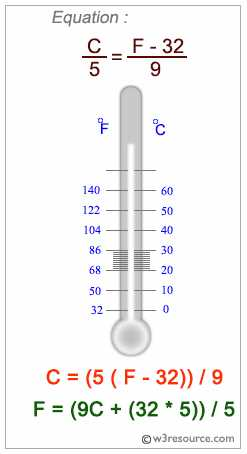When it comes to weather, temperature is often measured in degrees Celsius. However, if you’re more familiar with Fahrenheit or need to convert the temperature for a specific purpose, it’s important to know how to convert Celsius to Fahrenheit. Fortunately, the conversion formula is quite simple.

To convert Celsius to Fahrenheit, you can use the following formula:

Fahrenheit = (Celsius × 9/5) + 32

Here’s an example of how to use the formula:

Let’s say the temperature is 30 degrees Celsius. To convert it to Fahrenheit, you would use the formula:

Fahrenheit = (30 × 9/5) + 32

Simplifying the equation gives you:

Fahrenheit = (54) + 32

So, the temperature in Fahrenheit would be 86 degrees.

Here’s a table to help you convert Celsius to Fahrenheit for a range of temperatures:

Celsius Fahrenheit
-40 -40
-20 -4
0 32
10 50
20 68
30 86
40 104

Now you know how to convert Celsius to Fahrenheit. Whether you’re trying to understand the weather forecast or need to convert temperatures for a specific purpose, this knowledge will come in handy.

### Using the Formula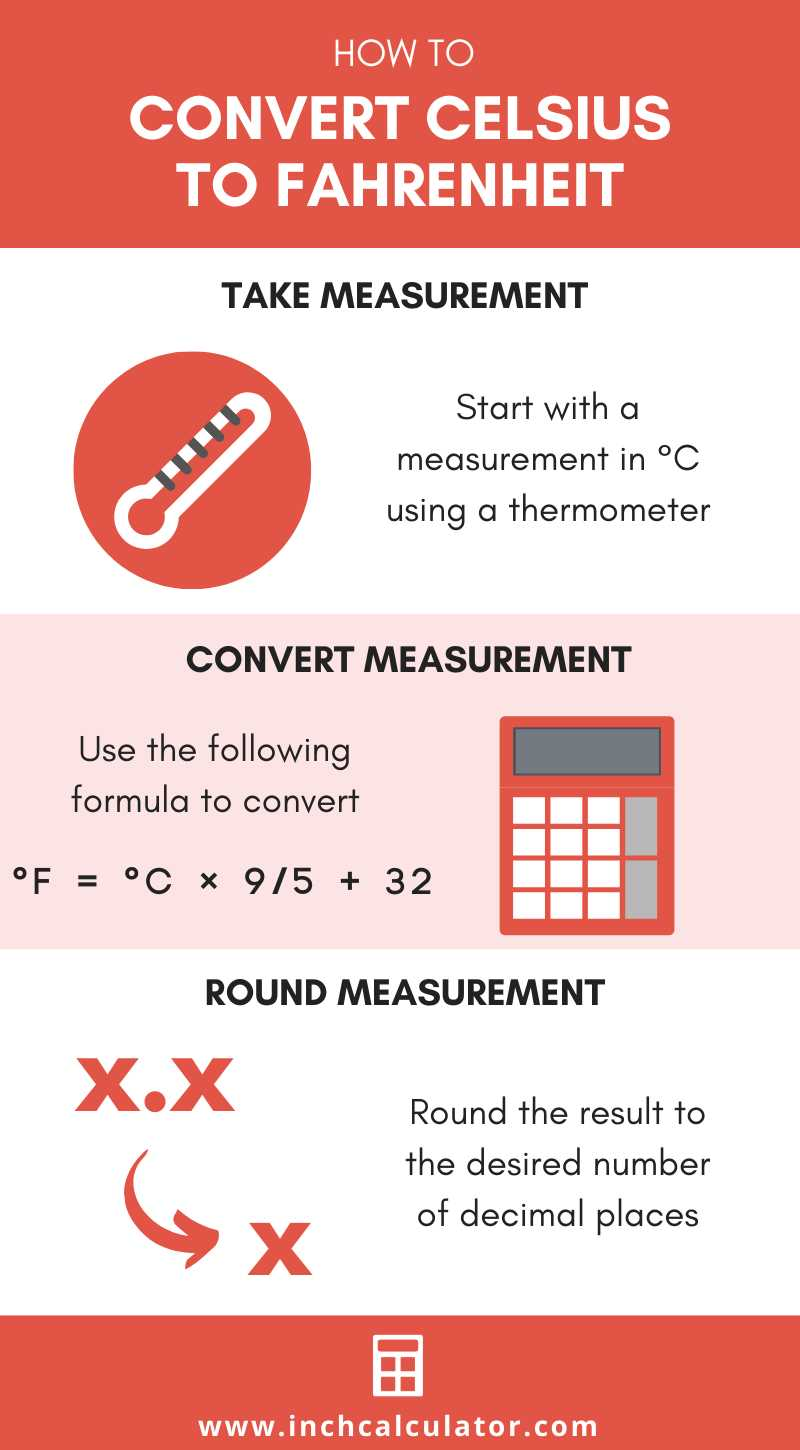To convert Celsius to Fahrenheit, you can use a simple formula:

1. Start by taking the temperature in degrees Celsius that you want to convert.
2. Multiply the Celsius temperature by 9/5.
3. Add 32 to the result.
4. The final number is the temperature in degrees Fahrenheit.

For example, let’s say you want to convert 30 degrees Celsius to Fahrenheit using the formula:

1. Multiply 30 by 9/5: 30 * 9/5 = 54
2. Add 32 to 54: 54 + 32 = 86

So, 30 degrees Celsius is equal to 86 degrees Fahrenheit.

This formula can be useful in various situations, such as when you want to convert the temperature from Celsius to Fahrenheit for weather reports, cooking recipes, or any other temperature conversion needs.

### Step-by-Step Conversion Process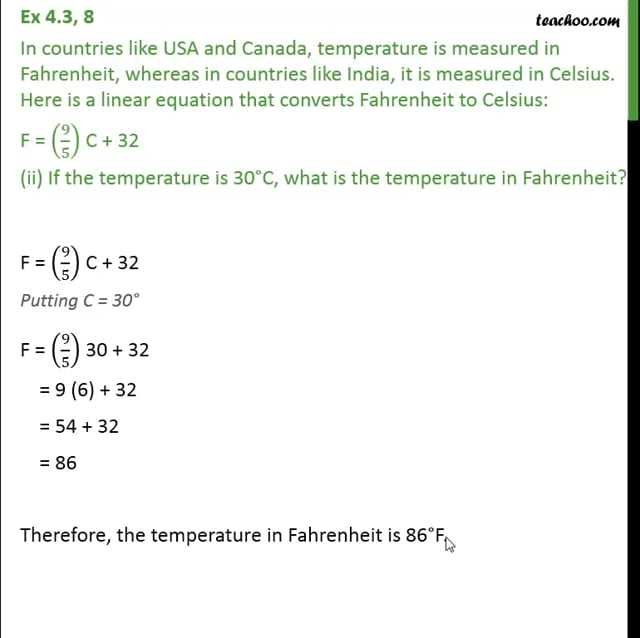To convert degrees Celsius to degrees Fahrenheit, you can follow these steps:

1. Start with the temperature in Celsius that you want to convert.
2. Multiply the Celsius temperature by 9/5.
3. Add 32 to the result from step 2.
4. The final result is the temperature in Fahrenheit.

To illustrate the conversion process, let’s convert 30 degrees Celsius to Fahrenheit using this formula:

Celsius Formula Fahrenheit
30 30 x 9/5 54
54 + 32 86

So, 30 degrees Celsius is equal to 86 degrees Fahrenheit.

If you find it difficult to perform the conversion manually, you can also use an online Celsius to Fahrenheit conversion calculator to get the accurate result.

### Converting Negative Celsius Temperatures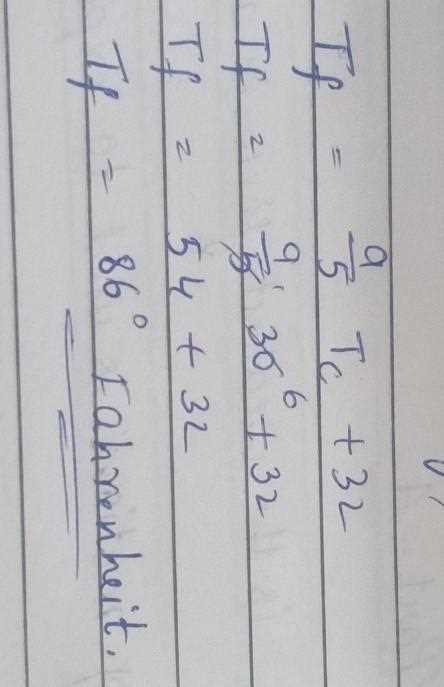When it comes to weather, temperatures can often drop below zero degrees Celsius. If you need to convert negative Celsius temperatures to Fahrenheit, the process is the same as converting positive temperatures, but with a few additional considerations.

Step 1: Start by determining the temperature in degrees Celsius that you want to convert. This could be a negative value, such as -10°C.

Step 2: Use the formula for converting Celsius to Fahrenheit: F = (C × 9/5) + 32. In this case, since the temperature is negative, make sure to include the negative sign in your calculations.

Step 3: Plug in the value of the temperature in degrees Celsius into the formula. For example, to convert -10°C to Fahrenheit, the calculation would be: F = (-10 × 9/5) + 32.

Step 4: Simplify the equation. In this case, the calculation would be: F = (-18) + 32.

Step 5: Complete the calculation. In this case, the final result would be: F = 14°F.

Step 6: Round the result to the nearest whole number, if desired. In this case, the final result of -10°C converted to Fahrenheit is approximately 14°F.

READ MORE  How to Choose the Perfect Twin Toddler Bed for Your Little Ones

Alternatively, you can use an online temperature conversion calculator or consult a temperature conversion table to quickly convert negative Celsius temperatures to Fahrenheit.

Remember, when dealing with negative Celsius temperatures, it’s important to include the negative sign in your calculations and keep track of any changes in the sign throughout the conversion process.

## Common Celsius to Fahrenheit Conversions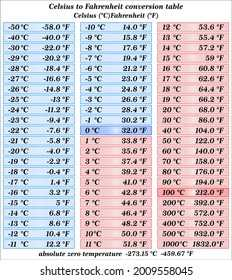Converting temperatures from Celsius to Fahrenheit is a common task when dealing with temperature measurements. Whether you’re checking the weather or using a temperature calculator, knowing how to convert between Celsius and Fahrenheit is important.

The formula to convert Celsius to Fahrenheit is:

Fahrenheit = Celsius × 9/5 + 32

Here are some common Celsius to Fahrenheit conversions:

Celsius Fahrenheit
0 degrees 32 degrees
10 degrees 50 degrees
20 degrees 68 degrees
30 degrees 86 degrees
40 degrees 104 degrees
50 degrees 122 degrees

Using a temperature conversion calculator or a conversion chart can also help you quickly convert Celsius to Fahrenheit. These tools are especially useful when you need to convert temperatures for scientific or engineering purposes.

Remember, Celsius and Fahrenheit are two different temperature scales, and knowing how to convert between them allows you to better understand and interpret temperature measurements.

Next time you come across a Celsius temperature, use the formula or a conversion tool to convert it to Fahrenheit and get a better sense of the temperature.